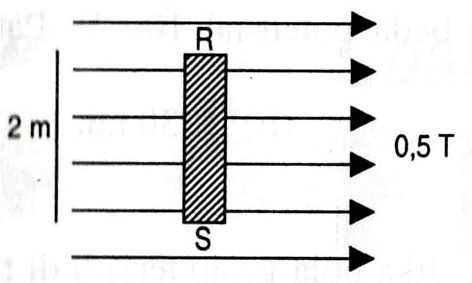# Magnetic force problem

## Homework StatementAs shown in figure above, a conductor RS with 2 m length which is powered with currents is put in a 0.5 T magnetic field. If the force generated by the conductor is 1 N into-the-screen, then the magnitude and direction of the current in conductor is ...

A. 1 A from R to S
B. 1 A from S to R
C. 2 A from R to S
D. 2 A from S to R
E. 5 A from R to S

F = BIL sin θ

## The Attempt at a Solution

1 = 0.5 I 2 sin 90°
I = 1 Ampere

And, the direction is from S to R
(I point my 4 fingers to the right side, my palm inward, so the thumb (current) points upward)

Is it correct?? I need to make sure about this... I'm self-studyingBvU
Homework Helper
Hi,

PF isn't really good at stamp-approving exercises -- it doesn't really help you if we do and you're found wrong anyway.
(It also antagonizes teachers in general).
You actually need to convince yourself that what you did is correct (because you fully understand what you did, checked it, etc.).

On the other hand, if I do the exercise I get the same result. I can't remember al these rules, but I can remember the Lorentz force $$\vec F_L = q\left (\vec E+\vec v\times\vec B\right )$$. And if I imagine a corkscrew and rotate ##\vec v## over the smallest angle to ##\vec B## I see that it goes into the page if ##\vec v## points from S to R

--

•terryds
Hi,

PF isn't really good at stamp-approving exercises -- it doesn't really help you if we do and you're found wrong anyway.
(It also antagonizes teachers in general).
You actually need to convince yourself that what you did is correct (because you fully understand what you did, checked it, etc.).

On the other hand, if I do the exercise I get the same result. I can't remember al these rules, but I can remember the Lorentz force $$\vec F_L = q\left (\vec E+\vec v\times\vec B\right )$$. And if I imagine a corkscrew and rotate ##\vec v## over the smallest angle to ##\vec B## I see that it goes into the page if ##\vec v## points from S to R

--

Alright, thanks..
Sometimes it's just hard to fully confident that it's 100% correct if I'm self-studying..
Thank you for your response anywayBvU
•